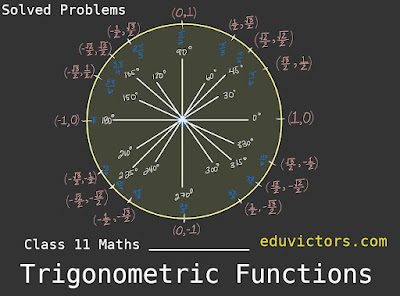# Class 11 - Maths - Trigonometric Functions Notes, Solved Problems and NCERT Exercise 3.2 Solutions

Answer: All angles which are integral multiples of π/2 are called quadrantal angles.

Therefore, for quadrantal angles, we have

cos 0° = 1,
sin 0° = 0,
cos $\frac{π}{2}$ = 0
sin $\frac{π}{2}$ = 1
cos π = − 1
sin π = 0
cos $\frac{3π}{2}$ = 0
sin $\frac{3π}{2}$ = -1
cos 2π = 1
sin 2π = 0

Trigonometric Functions

sin x = 0 implies x = nπ, where n is any integer
cos x = 0 implies x = (2n + 1) $\frac{π}{2}$, where n is any integer

Other Trigonometric Functions are:

cosec x = $\frac{1}{sin x}$, x ≠ nπ, where n is an integer

sec x  = $\frac{1}{cos x}$, x ≠ (2n + 1)$\frac{π}{2}$, where n is any integer

tan x = $\frac{sin x}{cos x}$, x ≠ (2n + 1)$\frac{π}{2}$, where n is any integer

cot x = $\frac{cos x}{sin x}$, ≠ nπ, where n is any integer

Trignometry Identies:

sin²θ + cos²θ = 1

sec²θ - tan²θ = 1

cosec²θ - cot²θ = 1

Trigonometric ratios of various angles:

(sin θ)(cosec θ) = 1, θ ≠ nπ, where n ∈ Z

(cos θ)(sec θ) = 1, θ ≠

Q2. Find the values of other five trigonometric functions if , cos x = $\frac{-1}{2}$ lies in third quadrant.

Answer:  cos x = $\frac{-1}{2}$

∴ sec x = $\frac{1}{cos x} = \frac{1}{\frac{-1}{2}}$

∴ sec x = -2

Since, sin²θ + cos²θ = 1

∴ sin²x = 1 - cos²x

sin²x = 1 - $(\frac{-1}{2})^{2}$

sin²x = 1 - $\frac{1}{4}$

sin²x = $\frac{3}{4}$

sin x = ±$\frac{\sqrt{3}}{2}$

Since x lies in the 3rd quadrant, the value of sin x will be negative

∴ sin x = -$\frac{\sqrt{3}}{2}$

cosec x = $\frac{1}{sin x}$

cosex x = $\frac{1}{-\frac{\sqrt{3}}{2}}$

cosec x = $\frac{2}{\sqrt{3}}$

tan x = $\frac{sin x }{cos x}$

tan x = $\frac{\frac{\sqrt{3}}{2}}{\frac{-1}{2}}$

tan x = $\sqrt{3}$

cot x = $\frac{1}{tan x}$

cot x = $\frac{1}{\sqrt{3}}$

Q3. Find the values of other five trigonometric functions if sin x = $\frac{3}{5}$, x lies in second quadrant.

Answer: sin x = $\frac{3}{5}$

cosec x = $\frac{1}{sin x} =\frac{1}{\frac{3}{5}}$

cosec x = $\frac{5}{3}$

Since, sin²x + cos²x = 1

cos²x  = 1 - sin²x

cos²x  = 1 - $(\frac{3}{5})^2$

cos²x  = 1 - $\frac{9}{25} = \frac{16}{25}$

cos x = ±$\frac{\sqrt{4}}{5}$

Since x lies in the 2nd quadrant, the value of cos x will be negative.

∴ cos x = -$\frac{\sqrt{4}}{5}$

sec x = $\frac{1}{cos x} = \frac{-5}{4}$

tan x = $\frac{sin x}{cos x} = \frac{-3}{4}$

cot x = $\frac{1}{tan x} = \frac{-4}{5}$

Q4. If $sin \theta = \frac{a- 4}{2}$ then a lies in ?

Answer: Since -1 ≤ sin θ ≤ 1
Thus we have,
-1 ≤ \frac{a-4}{2}$≤ 1 ⇒ -2 ≤ a - 4 ≤ 2 ⇒ 2 ≤ a ≤ 6 ⇒ a ∈ [2, 6] Important points you should know: (a) If A + B = 90° or 270°, then (i) sin²A + sin²B = 1 (ii) cos²A + cos²B = 1 (iii) tan A . tan B = 1 (iv) cot A . cot B = 1 (b) If A + B = 180°, then (i) cos A + cos B = 0 (ii) sin A - sin B = 0 (iii) tan A + tan B = 0 (c) If A + B = 360°, then (i) sin A + sin B = 0 (ii) cos A - cos B = 0 (iii) tan A + tan B = 0 Q5. sin²35 + sin²55 =? Answer: Since 35° + 55° = 90° ∴ sin²35 + sin²55 = 1 Q6. Find the value of the trigonometric function sin 765° Answer: We know that the values of sin x repeat after an interval of 2π or 360°. ∴ sin 765° = sin(2 × 360° + 45°) = sin 45° =$\frac{1}{\sqrt{2}}$Q7. Find the value of the trigonometric function cosec(–1410°) Answer: cosec(–1410°) = –cosec(1410°) We know that the values of cosec x repeat after an interval of 2π or 360°. ∴ -cosec(-1410°) = -cosec (4 × 360° - 30°) = cosec(30°) = 2 Q8. Find the value of the trigonometric function$\tan{\frac{19\pi}{3}}$Answer: We know that the values of tan x repeat after an interval of π or 180° Given$\tan{\frac{19\pi}{3}} = \tan{\6\pi + \frac{\pi}{3}}$=$\tan{\frac{\pi}{3}}$= tan 60° =$\sqrt{3}}\$